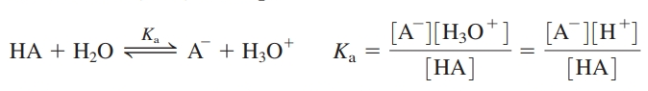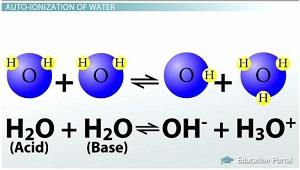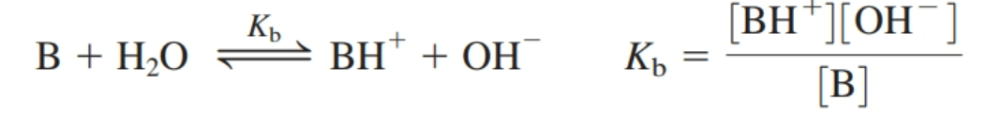# Exploring Chemical Analysis: Acid, Bases, and buffers. Flashcards

Set Details Share
created 3 days ago by Thomaslymanmiller
5 views
Definitions and equations
updated 1 day ago by Thomaslymanmiller
Subjects:
chemistry, science, life sciences, biophysics, analytic
Page to share:
Embed this setcancel
COPY
code changes based on your size selection
Size:
X

1

What is the definition of an acid?

A substance that increases the concentration of H+ when added to water.

2

What is the definition of an acid dissociation constant, KaThe equilibrium constant for the reacation of an acid, HA, with H2O

3

What is the definition of an acidic solution?

One in which the concentration of H+ is greater than the concentration of OH-

4

What is the definition of an amine?

A compound with the general formula RNH2, R2NH, or R3N, where R is any group of atoms

5

What is the definition of an ammonium ion?

The ammonium ion is NH4 +. An ammonium ion is any ion of the type RNH3 +, R2NH2+, R3NH+ , or R4N+ ,where R is an organic substituent.

6

What is the definition of autoprotolysis,Kw?The reaction in which two molecules of the same species transfer a proton from one to the other; Also called self-ionization.

7

What is the definition of a Base?

A substance that decreases the concentration of H+ when added to water.

8

What is the definition of a base hydrolysis constant, KbThe equilibrium constant for the reaction of a base, B, with H2O

9

What is the definition of a basic solution?

One in which the concentration of OH- is greater than the concentration of H+.

10

What is the definition of a carboxylate anion?

The conjugate base (RCO2 -) of a carboxylic acid.

11

What is the definition of a carboxylic acid?

A molecule with the general structure RCO2H, where R is any group of atoms.

12

What is the definition of a conjugate acid-base pair?

An acid and a base that differ only through the gain or loss of a single proton.

13

What is the definition of a hydronium ion,H3O+

What we really mean when we write H+(aq)

14

What is the definition of neutralization?

The process in which a stoichiometric equivalent of acid is added to a base (or vice versa).

15

What is the definition of pH?

Defined as pH =-log AH+ where AH+ is the activity of H+. In most approximate applications, the pH is taken as pH =-log[H+].

16

What is the definition of pK?

The negative logarithm (base 10) of an equilibrium constant: pK =-log K.

17

What is the definition of a salt?

An ionic solid.

18

What is the definition of a strong acid?

An acid that completely ionizes when dissolved in water is called a strong acid.

19

What is the definition of a strong base?

a base that completely ionizes when dissolved in water

20

What is the definition of a weak acid?

are acids that don't completely dissociate in solution.

21

What is the definition of a weak base?

are bases that don't completely dissociate in solution.

22

What is the definition of a buffer?

A mixture of a weak acid and its conjugate base. A buffered solution is one that resists changes in pH when acids or bases are added

23

What is the definition of an indicator?

A compound having a physical property (usually color) that changes abruptly near the equivalence point of a chemical reaction.

24

What is the Brønsted and Lowry definition of an acid and a base?

an acid is a proton donor and a base is a proton acceptor.

25

What is HCl and is it a strong acid or base?

Hydrochloric acid" (hydrogen chloride) and it's a strong acid.

26

Is HBr (Hydrogen bromide) a strong acid or base?

It is a strong acid.

27

Is HI (Hydrogen Iodide) a strong acid or base?

It is a strong acid.

28

What is H2SO4 and is it a strong acid or base?

Sulfuric acid and It is a strong acid.

29

What is HNO3 and is ita strong acid or base?

Nitric acid and it is a strong acid.

30

What is HClO4 and is it a strong acid or base?

Perchloric acid and It is a strong acid.

31

Is LiOH (lithium hydroxide) a strong acid or base?

It is a strong base.

32

Is NaOH (sodium hydroxide) a strong acid or base?

It is a strong base.

33

Is KOH (potassium hydroxide) a strong acid or base?

It is a strong base.

34

Is RbOH (rubidium hydroxide) a strong acid or base?

It is a strong base.

35

Is CsOH (cesium hydroxide) a strong acid or base?

It is a strong base.

36

What is R4NOHb and is it a strong acid or base?

It quaternary ammonium hydroxide and it is a strong base.